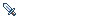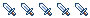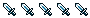## Error: Could not find map file (gentoo ebuild)

Ask for help regarding any technical issue or report any bug or OS independent issues.
flamma
PeonPosts: 10
Joined: 30 Dec 2005, 01:23

### Error: Could not find map file (gentoo ebuild)

I get this error since I-don't-remember-which version, so I can't play.

Code: Select all

``````me@mymachine ~ \$ tmw
The Mana World v0.0.18
Error: Could not find map file
me@mymachine ~ \$
``````
What can I do to continue playing?
Platyna
Former DictatorPosts: 2216
Joined: 19 Nov 2005, 14:19
Location: Poland
Contact:
See the logfile of TMW for more infor and paste it here.

Regards.
Zuzanna K. Filutowska
[The rest of this signature was censored by TMWC's "Freedom" and "Free speech".]
flamma
PeonPosts: 10
Joined: 30 Dec 2005, 01:23
The last lines (previous lines doesn't seem very important in this case).

Code: Select all

``````[00:19:13] Error: Unknown error while decompressing map data!
[00:19:13] Error: Could not find map file
``````
Platyna
Former DictatorPosts: 2216
Joined: 19 Nov 2005, 14:19
Location: Poland
Contact:
Is there everything ok with your gzip and zlib? Paste strace.

Regards.
Zuzanna K. Filutowska
[The rest of this signature was censored by TMWC's "Freedom" and "Free speech".]
Bjørn
TMW AdviserPosts: 1430
Joined: 09 Dec 2004, 19:50
Location: North Rhine-Westphalia, Germany
Contact:
Are you using Gentoo?
flamma
PeonPosts: 10
Joined: 30 Dec 2005, 01:23
Yes, I am using Gentoo. Sorry, I wrote it in the subject, but forgot to write it in the message. Are there known problems with Gentoo?

Here I put the las few lines of strace (since the moment I think tmw tries to load the map):

Code: Select all

``````mmap2(NULL, 2461696, PROT_READ|PROT_WRITE, MAP_PRIVATE|MAP_ANONYMOUS, -1, 0) = 0xb6052000
mmap2(NULL, 2461696, PROT_READ|PROT_WRITE, MAP_PRIVATE|MAP_ANONYMOUS, -1, 0) = 0xb59a7000
munmap(0xb59a7000, 2461696)             = 0
mmap2(NULL, 2461696, PROT_READ|PROT_WRITE, MAP_PRIVATE|MAP_ANONYMOUS, -1, 0) = 0xb59a7000
munmap(0xb6052000, 2461696)             = 0
open("/home/pablo//.tmw/updates/update-0.0.17-0.0.18.zip", O_RDONLY) = 13
lseek(13, 0, SEEK_SET)                  = 0
read(13, "PK\3\4", 4)                   = 4
read(13, "\24\0", 2)                    = 2
read(13, "\0\0", 2)                     = 2
read(13, "\10\0", 2)                    = 2
read(13, ",\275\2153", 4)               = 4
read(13, "\356\31\233\243", 4)          = 4
read(13, "\226\7\0\0", 4)               = 4
read(13, "\265\'\0\0", 4)               = 4
read(13, "\t\0", 2)                     = 2
read(13, "\25\0", 2)                    = 2
lseek(13, 60, SEEK_SET)                 = 60
read(13, "\305\232\333\216\3336\20\206\257\223\247`\f\24\271\330"..., 1942) = 1942
close(13)                               = 0
open("/home/pablo//.tmw/updates/update-0.0.17-0.0.18.zip", O_RDONLY) = 13
lseek(13, 486461, SEEK_SET)             = 486461
read(13, "PK\3\4", 4)                   = 4
read(13, "\24\0", 2)                    = 2
read(13, "\0\0", 2)                     = 2
read(13, "\10\0", 2)                    = 2
read(13, "\317\21m3", 4)                = 4
read(13, "\"C6\342", 4)                 = 4
read(13, "`\35\0\0", 4)                 = 4
read(13, "Y\37\0\0", 4)                 = 4
read(13, "\23\0", 2)                    = 2
read(13, "\25\0", 2)                    = 2
lseek(13, 486531, SEEK_SET)             = 486531
read(13, "\245xyXR]\333/Y6Y\221\231Q\251\320\370hZRV\217#P\16\331"..., 7520) = 7520
close(13)                               = 0
mmap2(NULL, 266240, PROT_READ|PROT_WRITE, MAP_PRIVATE|MAP_ANONYMOUS, -1, 0) = 0xb67fa000
time()                      = 1135940929
write(8, "[11:08:49] Error: Unknown error "..., 62) = 62
time()                      = 1135940929
write(8, "[11:08:49] Error: Could not find"..., 42) = 42
write(2, "E", 1E)                        = 1
write(2, "r", 1r)                        = 1
write(2, "r", 1r)                        = 1
write(2, "o", 1o)                        = 1
write(2, "r", 1r)                        = 1
write(2, ":", 1:)                        = 1
write(2, " ", 1 )                        = 1
write(2, "C", 1C)                        = 1
write(2, "o", 1o)                        = 1
write(2, "u", 1u)                        = 1
write(2, "l", 1l)                        = 1
write(2, "d", 1d)                        = 1
write(2, " ", 1 )                        = 1
write(2, "n", 1n)                        = 1
write(2, "o", 1o)                        = 1
write(2, "t", 1t)                        = 1
write(2, " ", 1 )                        = 1
write(2, "f", 1f)                        = 1
write(2, "i", 1i)                        = 1
write(2, "n", 1n)                        = 1
write(2, "d", 1d)                        = 1
write(2, " ", 1 )                        = 1
write(2, "m", 1m)                        = 1
write(2, "a", 1a)                        = 1
write(2, "p", 1p)                        = 1
write(2, " ", 1 )                        = 1
write(2, "f", 1f)                        = 1
write(2, "i", 1i)                        = 1
write(2, "l", 1l)                        = 1
write(2, "e", 1e)                        = 1
write(2, "\n", 1
)                       = 1
rt_sigprocmask(SIG_SETMASK, NULL, [RTMIN], 8) = 0
rt_sigsuspend([] <unfinished ...>
--- SIGRTMIN (Unknown signal 32) @ 0 (0) ---
<... rt_sigsuspend resumed> )           = -1 EINTR (Interrupted system call)
sigreturn()                             = ? (mask now [RTMIN])
write(7, "\240\26\314\266\1\0\0\0\2@\0\0@F\374\267\3\0\0\0\0\0\0"..., 148) = 148
rt_sigprocmask(SIG_SETMASK, NULL, [RTMIN], 8) = 0
rt_sigsuspend([] <unfinished ...>
--- SIGRTMIN (Unknown signal 32) @ 0 (0) ---
<... rt_sigsuspend resumed> )           = -1 EINTR (Interrupted system call)
sigreturn()                             = ? (mask now [RTMIN])
write(7, "\240\26\314\266\1\0\0\0\3\200\0\0<\224\337\267\0\0\0\0"..., 148) = 148
close(9)                                = 0
write(4, "_\3\2\0\v\0 \3+\0\1\0", 12)   = 12
read(4, "\1\2\177\0\0\0\0\0.\0\0\3\0\0\0\0\0\0\0\0\5\0\0\0p\311"..., 32) = 32
write(5, "+\3\1\0", 4)                  = 4
read(5, "\1\2\375\f\0\0\0\0.\0\0\3\0\0\0\0\1\0\0\0\6\0\0\0HDq\10"..., 32) = 32
write(4, "\223\2\2\0\23\0 \3+\0\1\0", 12) = 12
read(4, "\1\2\201\0\0\0\0\0.\0\0\3\0\0\0\0\0\0\0\0\5\0\0\0p\311"..., 32) = 32
shmdt(0xb6891000)                       = 0
write(4, "\n\2\2\0\3\0 \3\4\0\2\0\21\0 \3<\0\2\0\22\0 \3O\0\2\0\20"..., 44) = 44
read(4, "\22\0\202\0\3\0 \3\3\0 \3\0@q\10\3\0 \3\0\0\0\0\370\'\223"..., 32) = 32
read(4, "\1\2\207\0\0\0\0\0.\0\0\3\0\0\0\0\0\0\0\0\5\0\0\0p\311"..., 32) = 32
write(5, "<\3\2\0\0\0@\3+\0\1\0", 12)   = 12
read(5, "\1\2\377\f\0\0\0\0.\0\0\3\0\0\0\0\0\0\0\0\6\0\0\0HDq\10"..., 32) = 32
shutdown(5, 2 /* send and receive */)   = 0
close(5)                                = 0
write(4, "<\2\2\0\0\0 \3+\0\1\0", 12)   = 12
read(4, 0xbfdc0570, 32)                 = -1 EAGAIN (Resource temporarily unavailable)
select(5, , NULL, NULL, NULL)        = 1 (in )
read(4, "\1\2\211\0\0\0\0\0.\0\0\3\0\0\0\0\0\0\0\0\5\0\0\0p\311"..., 32) = 32
shutdown(4, 2 /* send and receive */)   = 0
close(4)                                = 0
rt_sigaction(SIGSEGV, NULL, {0xb7d21e60, [], 0}, 8) = 0
rt_sigaction(SIGSEGV, {SIG_DFL}, NULL, 8) = 0
rt_sigaction(SIGBUS, NULL, {0xb7d21e60, [], 0}, 8) = 0
rt_sigaction(SIGBUS, {SIG_DFL}, NULL, 8) = 0
rt_sigaction(SIGFPE, NULL, {0xb7d21e60, [], 0}, 8) = 0
rt_sigaction(SIGFPE, {SIG_DFL}, NULL, 8) = 0
rt_sigaction(SIGQUIT, NULL, {0xb7d21e60, [], 0}, 8) = 0
rt_sigaction(SIGQUIT, {SIG_DFL}, NULL, 8) = 0
getpid()                                = 7879
getpid()                                = 7879
getpid()                                = 7879
getpid()                                = 7879
munmap(0xb6c66000, 372736)              = 0
munmap(0xb7fab000, 8192)                = 0
write(7, "\240\26\314\266\2\0\0\0\1\0\0\0\0@\250\267PJ\250\267\10"..., 148) = 148
rt_sigprocmask(SIG_SETMASK, NULL, [RTMIN], 8) = 0
rt_sigsuspend([] <unfinished ...>
--- SIGRTMIN (Unknown signal 32) @ 0 (0) ---
<... rt_sigsuspend resumed> )           = -1 EINTR (Interrupted system call)
sigreturn()                             = ? (mask now [RTMIN])
waitpid(7880, NULL, __WCLONE)           = 7880
munmap(0xb7f94000, 4096)                = 0
munmap(0xb7faa000, 4096)                = 0
exit_group(1)                           = ?
``````
Bjørn
TMW AdviserPosts: 1430
Joined: 09 Dec 2004, 19:50
Location: North Rhine-Westphalia, Germany
Contact:
flamma wrote:Yes, I am using Gentoo. Sorry, I wrote it in the subject, but forgot to write it in the message. Are there known problems with Gentoo?
Yes this is an already reported problem by another Gentoo user.

Topic: Bug: Unknown error while decompressing map data

Sorry the strace doesn't seem very useful to me. Topic locked, discussion can continue in the earlier thread.